Vol. DIY Electronics Projects
Chapter 3 DC Circuit Projects

# DC Lab - Parallel Batteries

## In this hands-on electronics experiment, you will connect batteries in parallel to power a light and learn the relationship between the individual battery currents and the total system current.

### Project Overview

This experiment aims to explore the effect of connecting multiple batteries in parallel to increase the current and light intensity of a lamp. Connecting identical batteries in parallel, as shown in Figure 1, means connecting them so that all of the negative terminals are connected together, and all of the positive terminals are connected together.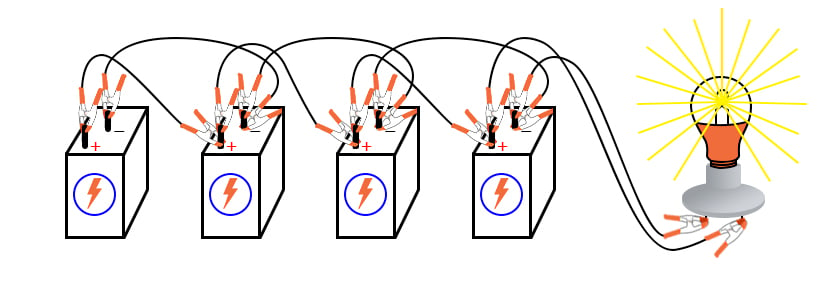##### Figure 1. Connecting four batteries in parallel to power a light.

When you connect batteries in parallel, you will find that the system voltage is the same as the individual battery voltages, but the total current that can be supplied to a load, like the light shown in this figure, increased. A circuit schematic representation of the four batteries connected in parallel to power a light source is illustrated in Figure 2.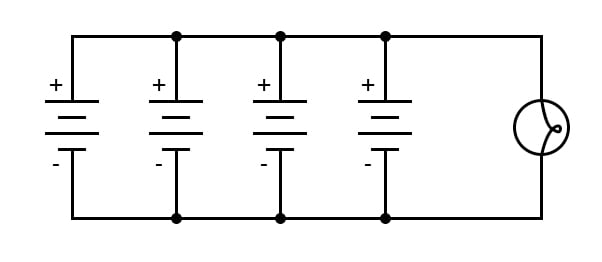### Parts and Materials

• Four 6 V batteries
• 12 V light bulb, 25 or 50 W
• Light socket

High-wattage 12 V lamps may be purchased from recreational vehicles (RVs) and boating supply stores. They are becoming increasingly difficult to find as lighting changes from incandescent to LEDs. Common sizes are 25 W and 50 W. This lamp will be used as a “heavy” load for your batteries, where a heavy load is defined as one that draws substantial current. A regular household (120 V) lamp socket will work just fine for these low-voltage RV lamps.

### Instructions

Step 1: The initial step is to connect a 6 V battery to the light, which is designed to operate on 12 volts, as shown in Figure 3. The lamp should glow dimly when powered by the 6 V battery since the insufficient voltage is being applied.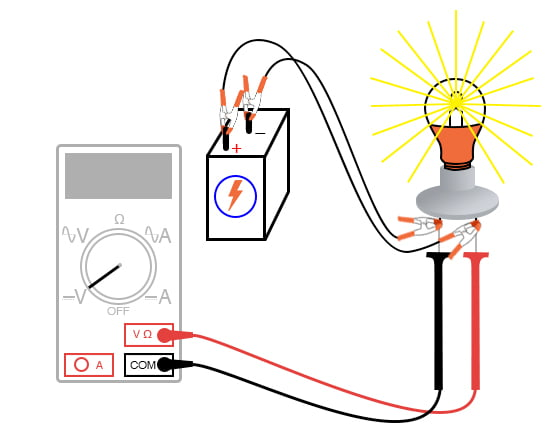##### Figure 3. Measuring the lamp voltage.

Step 2: Next, measure the voltage across the lamp with a voltmeter. It may be lower than 6 V. This is because the high-power lamp draws a large current, causing the voltage at the battery terminals to drop or "sag" due to the internal resistance of the battery.

Step 3: To overcome this problem, we will add more batteries in parallel so that each battery only has to supply a fraction of the total current required by the lamp. To make a parallel connection, the positive terminals of all the batteries are connected together, and the negative terminals are connected together, as shown in Figure 4. Add one battery at a time, and then note the intensity of the lamp and measure the voltage at the lamp. The light intensity should increase as the voltage sag is reduced.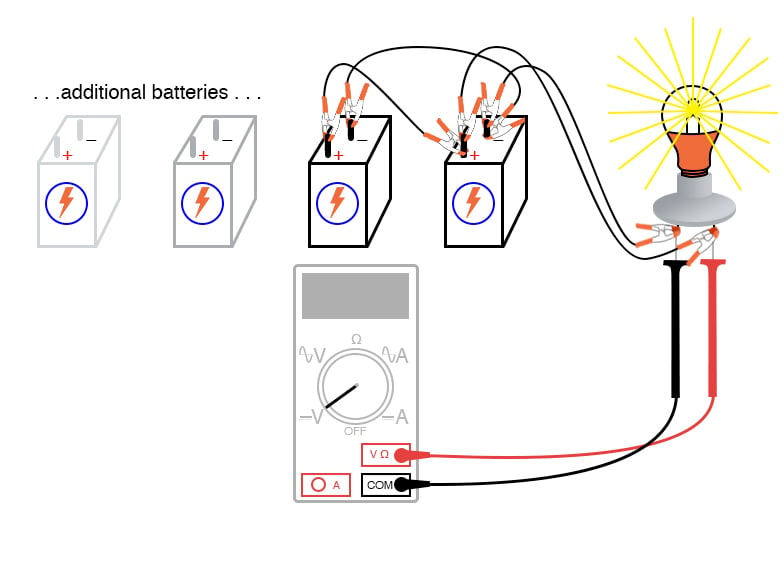##### Figure 4. Using a voltmeter to measure the lamp voltage with parallel battery sources.

Step 4: To measure the current of a single battery, the circuit for one battery is broken, and an ammeter is inserted within that break to measure the current of that one battery (Figure 5).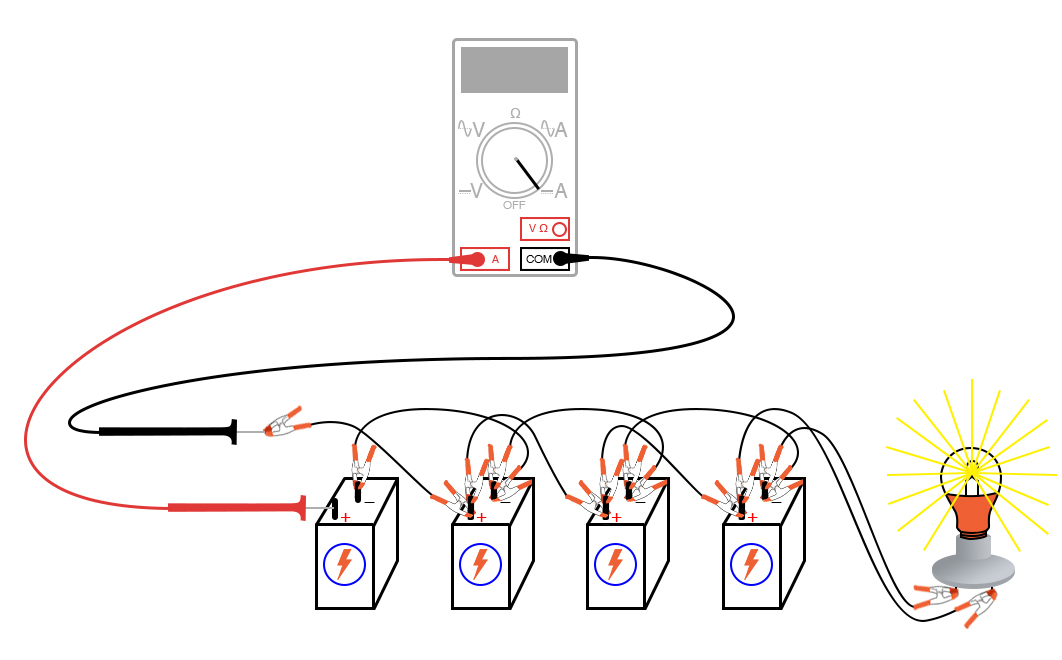##### Figure 5. Using an ammeter to measure the current from a single battery.

Step 5: Measuring the total current involves a similar procedure by breaking the circuit and inserting the ammeter, as shown in Figure 6. The difference in current between the single battery and total measurements should be noted.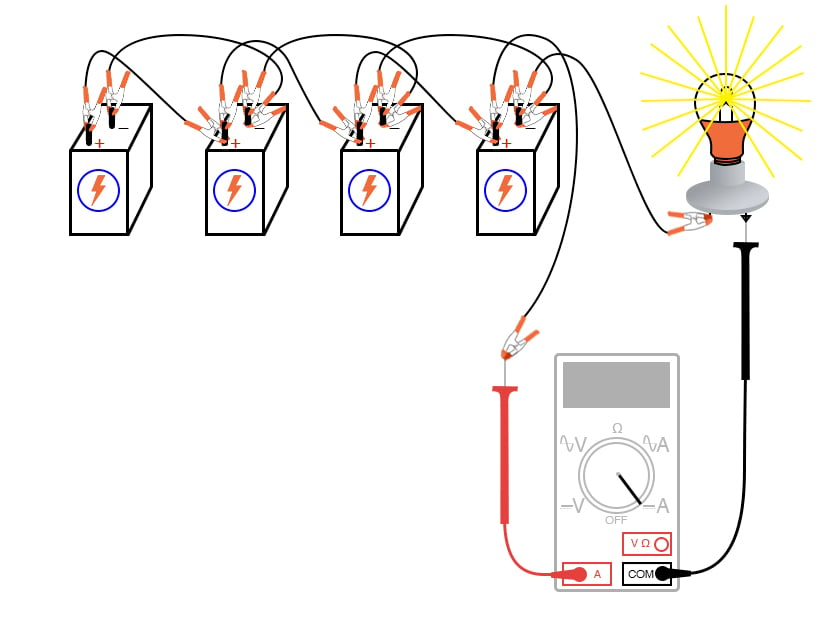##### Figure 6. Using an ammeter to measure the total current from four batteries in parallel.

Step 6: Finally, to achieve the maximum brightness from the light bulb, connect four 6 V batteries in a series-parallel connection. As we demonstrated in the series battery experiment, connecting two 6 V batteries in series will provide 12 V. Now, connecting two of these series-connected battery pairs in parallel, as shown in Figure 7, improves their current-sourcing ability and minimizes voltage sag.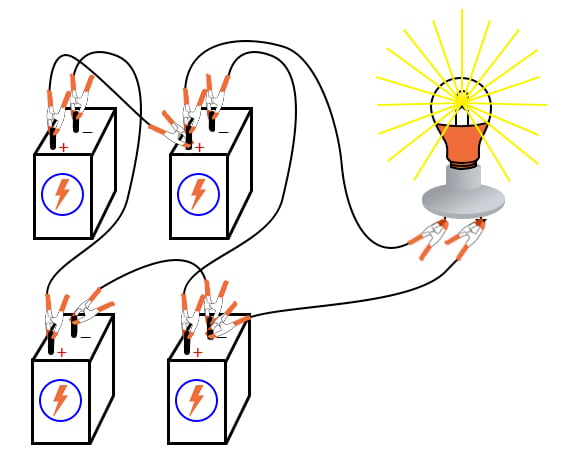### Related Content

Calculators:

Textbook Pages:

Worksheets:

Video Tutorials and Lectures:

#### Lessons in Electric Circuits

Published under the terms and conditions of the Design Science License